Normal view

# Regression for categorical data

Publisher: Cambridge Cambridge University Press 2012Description: x, 561 pISBN: 1107009650ISSN: 9780511842061DDC classification: 519.536 Online resources: E-Book Summary: This book introduces basic and advanced concepts of categorical regression with a focus on the structuring constituents of regression, including regularization techniques to structure predictors. In addition to standard methods such as the logit and probit model and extensions to multivariate settings, the author presents more recent developments in flexible and high-dimensional regression, which allow weakening of assumptions on the structuring of the predictor and yield fits that are closer to the data. A generalized linear model is used as a unifying framework whenever possible in particular parametric models that are treated within this framework. Many topics not normally included in books on categorical data analysis are treated here, such as nonparametric regression; selection of predictors by regularized estimation procedures; ternative models like the hurdle model and zero-inflated regression models for count data; and non-standard tree-based ensemble methods, which provide excellent tools for prediction and the handling of both nominal and ordered categorical predictors. The book is accompanied an R package that contains data sets and code for all the examples.
List(s) this item appears in: VR_VSL e-Book collection
Tags from this library: No tags from this library for this title.Average rating: 0.0 (0 votes)
Item type Current location Collection Call number Status Date due Barcode
eBooks Vikram Sarabhai Library
Electronic Resources
Non-fiction 519.536 T8R3 (Browse shelf) Available ER000498
##### Browsing Vikram Sarabhai Library shelves, Shelving location: Electronic Resources, Collection: Non-fiction Close shelf browser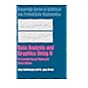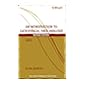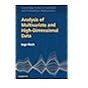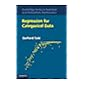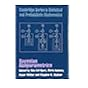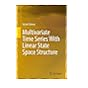519.50285 M2D2 Data analysis and graphics using R: an example-based approach 519.535 A4I6/2007 Introduction to categorical data analysis 519.535 K6A6 Analysis of multivariate and high-dimensional data 519.536 T8R3 Regression for categorical data 519.542 B2 Bayesian nonparametrics 519.55 G6M8 Multivariate time series with linear state space structure 615.19 Bayesian analysis with R for drug development: concepts, algorithms, and case studies

1. Introduction
2. Binary Regression: The Logit Model
3. Generalized Linear Models
4. Modeling of Binary Data
5. Alternative Binary Regression Models
6. Regularization and Variable Selection for Parametric Models
7. Regression Analysis of Count Data
8. Multinomial Response Models
9. Ordinal Response Models
10. Semi- and Non-Parametric Generalized Regression
11. Tree-Based Methods
12. The Analysis of Contingency Tables: Log-Linear and Graphical Models
13. Multivariate Response Models
14. Random Effects Models and Finite Mixtures
15. Prediction and Classification

This book introduces basic and advanced concepts of categorical regression with a focus on the structuring constituents of regression, including regularization techniques to structure predictors. In addition to standard methods such as the logit and probit model and extensions to multivariate settings, the author presents more recent developments in flexible and high-dimensional regression, which allow weakening of assumptions on the structuring of the predictor and yield fits that are closer to the data. A generalized linear model is used as a unifying framework whenever possible in particular parametric models that are treated within this framework. Many topics not normally included in books on categorical data analysis are treated here, such as nonparametric regression; selection of predictors by regularized estimation procedures; ternative models like the hurdle model and zero-inflated regression models for count data; and non-standard tree-based ensemble methods, which provide excellent tools for prediction and the handling of both nominal and ordered categorical predictors. The book is accompanied an R package that contains data sets and code for all the examples.

There are no comments for this item.